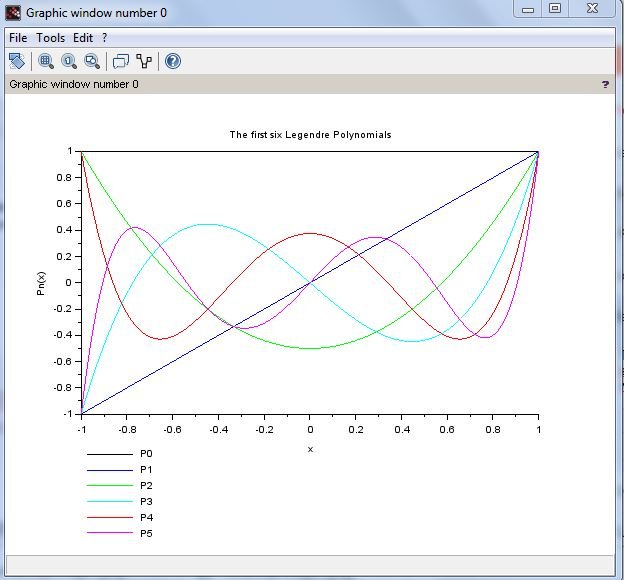To find Legendre Polynomial using scilab:

AIM OF THE EXPERIMENT:
To find Legendre Polynomial using scilab
REQUISITES:
A PC/laptop.
Scilab 6.0.0 must be installed.
THEORY:
The Legendre differential equation is the second-order ordinary differential equationTo evaluate Legendre polynomial in scilab we use legendre(n,m,x) which is the associated Legendre polynomial. However we need one Legendre polynomial, so let m=0.
Let us find & plot 1st six Legendre polynomial for x=-1 to x=+1.
PROCEDURE:
2. Go to all programs & open scilab 6.0.0.
3. Go to Scinotes.
4. Write the coding/program.
5. Save the file & use extension name .sci
6. Then execute & go to the scilab console window for output.
7. Go to the graphic window to get the graph which is the output.
CODING:
```clc;
clf;
n=0:5;
x=[-1:0.001:1]';
y=legendre(n,0,x)';
plot2d(x,y,leg='P0@P1@P2@P3@P4@P5');
xtitle('The first six Legendre Polynomials')
ylabel('Pn(x)');
xlabel('x');```
```
OUTPUT:Scilab Programming

C ++ Programming

Physics Notes: CLICK

IIT-JAM             JEST                GATE               NET